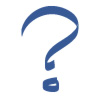I have forgotten

•http://facebook.com/
•https://www.google.com/accounts/o8/id
•https://me.yahoo.com

# Unbalanced Eqn23 Apr 20, 3:37AM
Unbalanced Eqn
Below my equation, it showing ' Unbalanced Eqn '

&{|l|l|l|}
Figure/Alphabet & Line of symmetry \
{Rectangle} &
{2}\
{Equilateral triangle} &
{3}\
A &
{1}\
R &
{0}\
{Square} &
{4}\

I dont know why, please let me know if i am doing any mistake and also the above equation i wrapped in img tag <img src="http://latex.codecogs.com/svg.latex? " border="0"/> like this <img src="http://latex.codecogs.com/svg.latex?

&{|l|l|l|}
Figure/Alphabet & Line of symmetry \
{Rectangle} &
{2}\
{Equilateral triangle} &
{3}\
A &
{1}\
R &